# To write three similarity statements about the triangles and the reason that they are similar. Given that 12210203151.jpgQuestion
SimilarityTo write three similarity statements about the triangles and the reason that they are similar.
Given that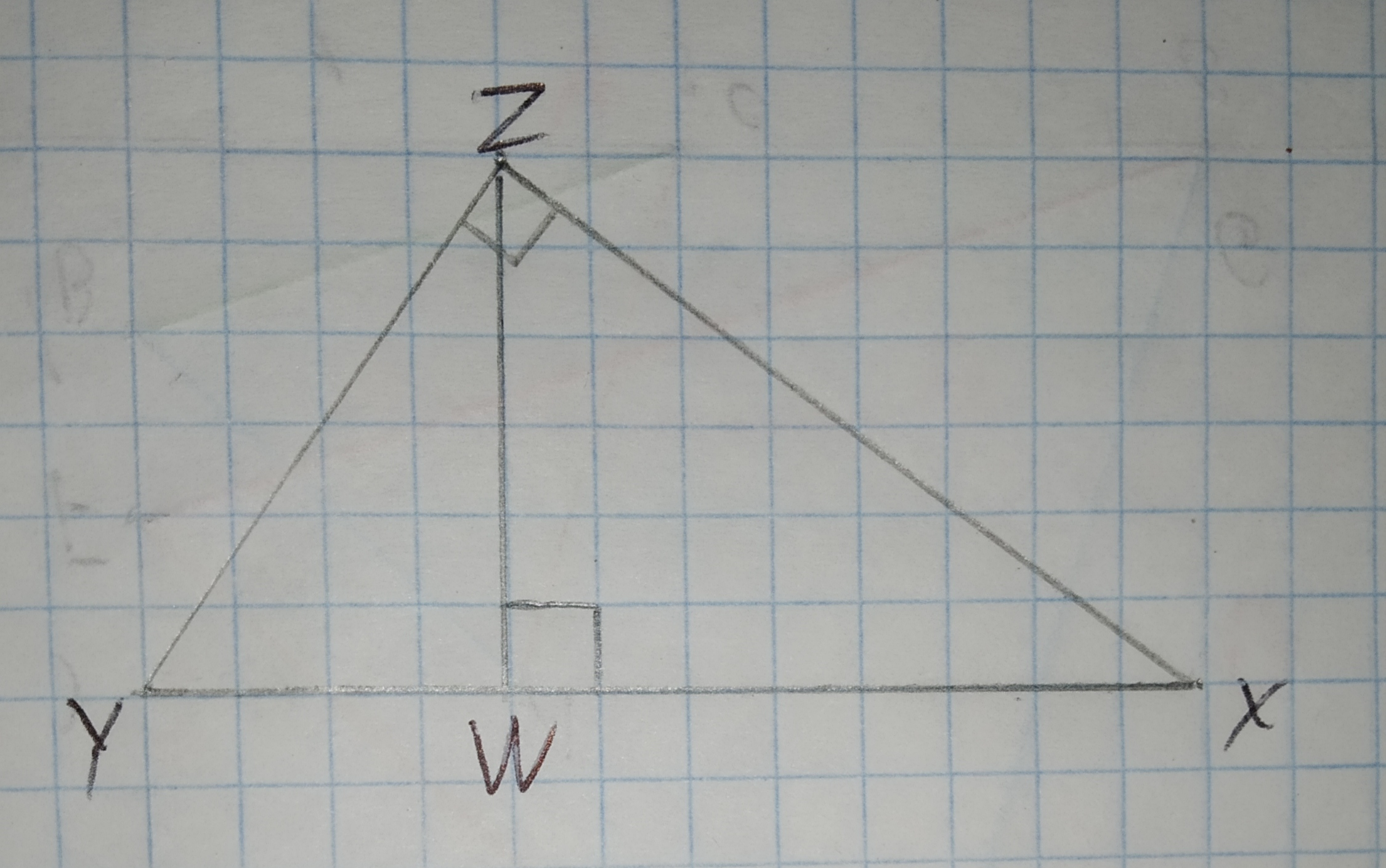2021-01-08
The triangle XYZ and the triangles XWZ and YWZ are right triangles.
Since,
$$\displaystyle\angle{Z}{W}{X}=\angle{Y}{Z}{X}=\angle{Y}{Z}{W}={90}^{{\circ}}$$
Also, midpoint of line XY divide the base proportionally.
Therefore, the sides are also proportional.
Therefore, by the rules of similarity, the triangles are proportional.

### Relevant QuestionsGiven $$\displaystyle\triangle{D}{E}{F}{\quad\text{and}\quad}\triangle{E}{G}{F}$$ in the diagram below, determine if the triangles are similar. If so, write a similarity statement, and state the criterion used to support your claim.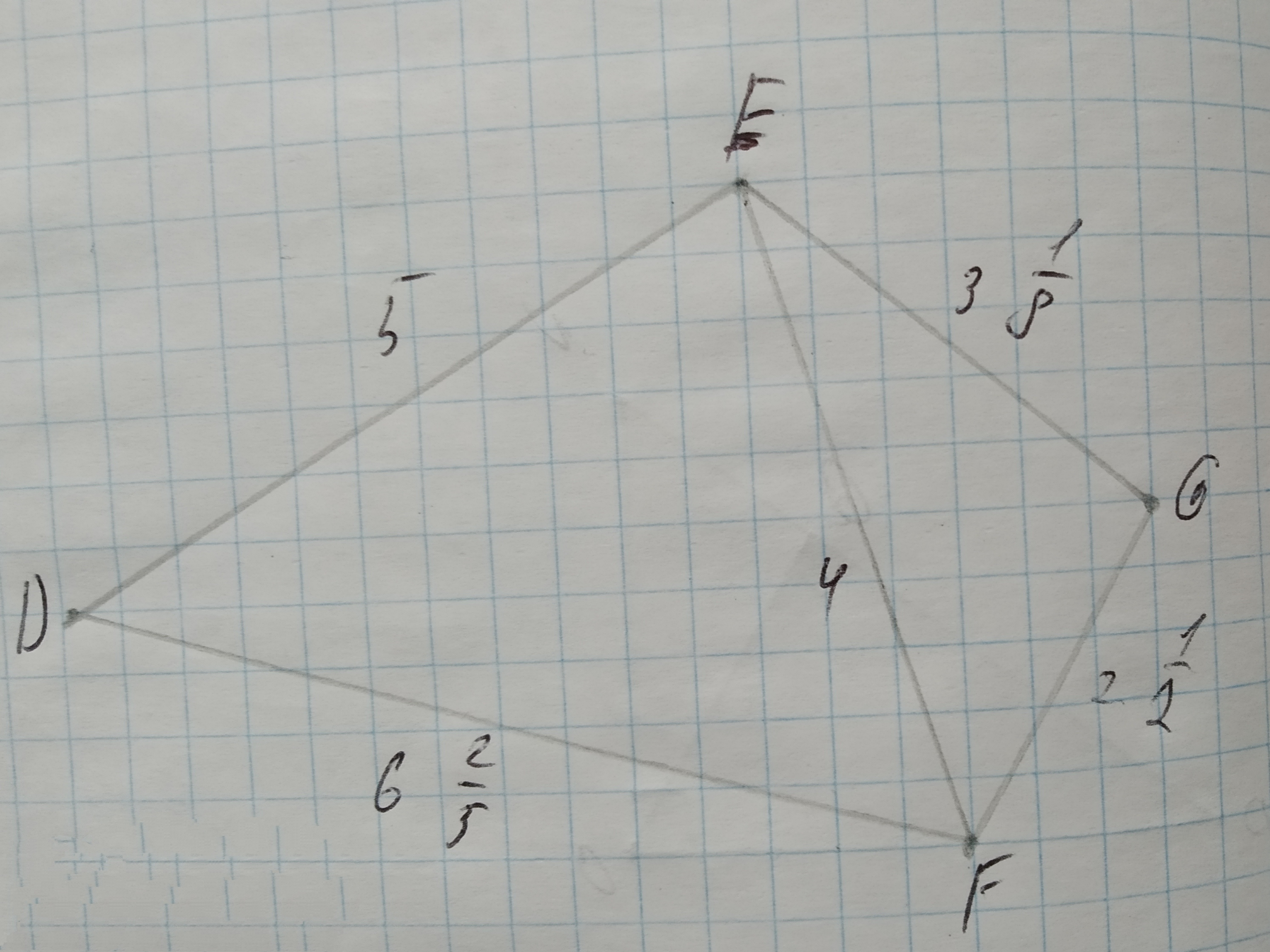To check: whether the triangles are similar. If so, write a similarity statement.
Given:
The given triangles are: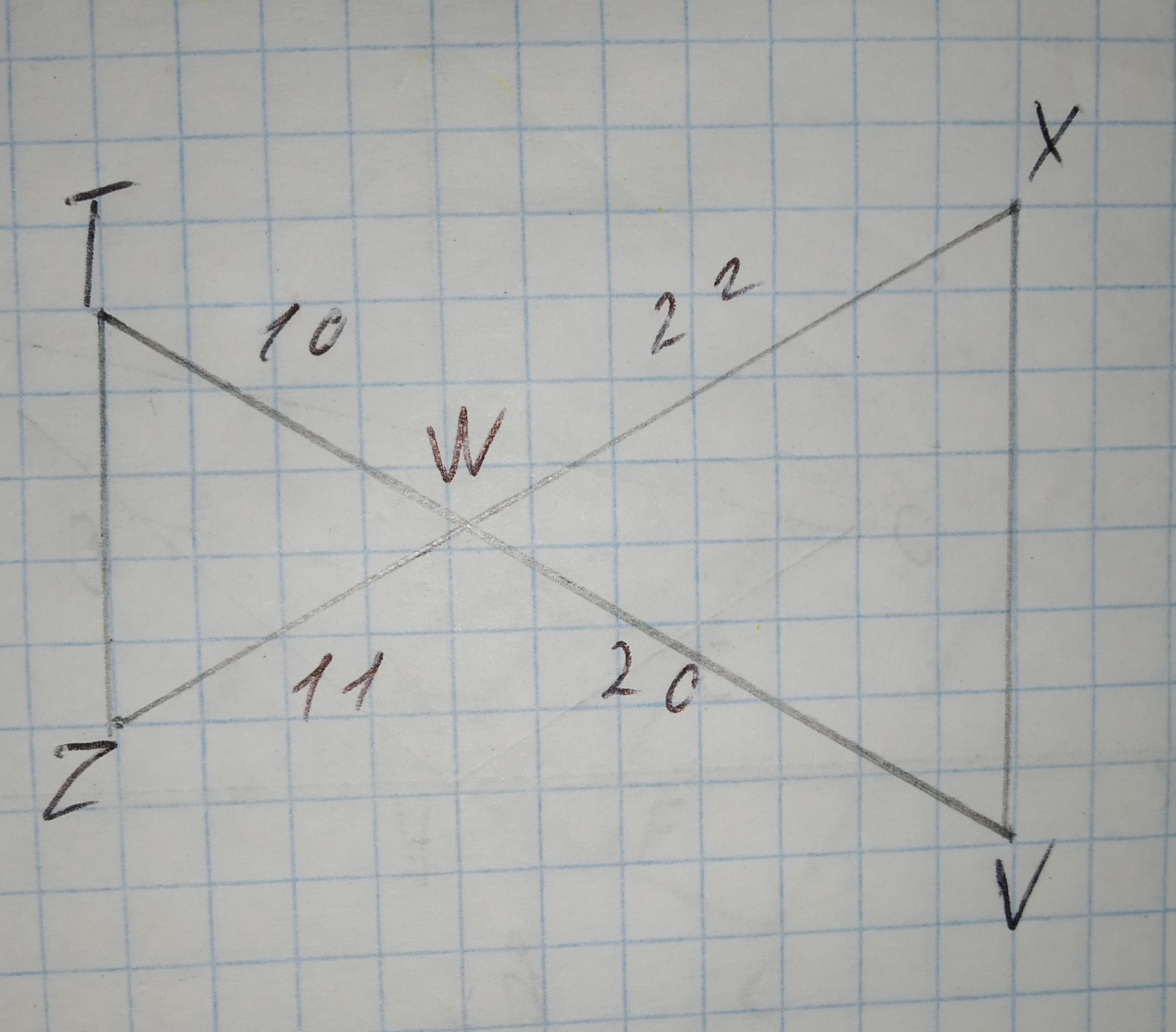To determine:Given triangles are similar or not, if not give reason.
Given information: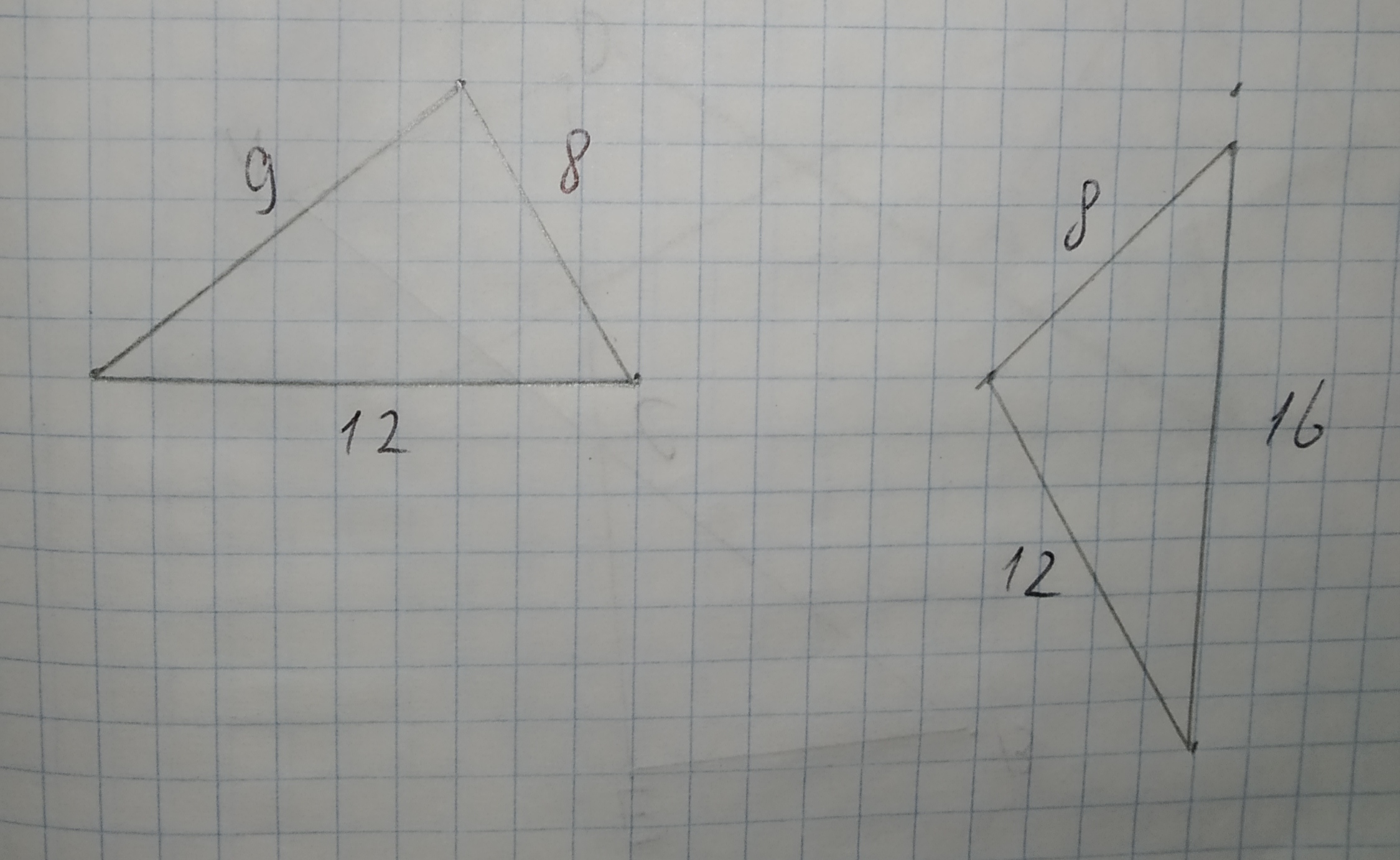Determine whether the triangles are similar.
If so, write a similarity statement and name the postulate or theorem you used. If not, explain.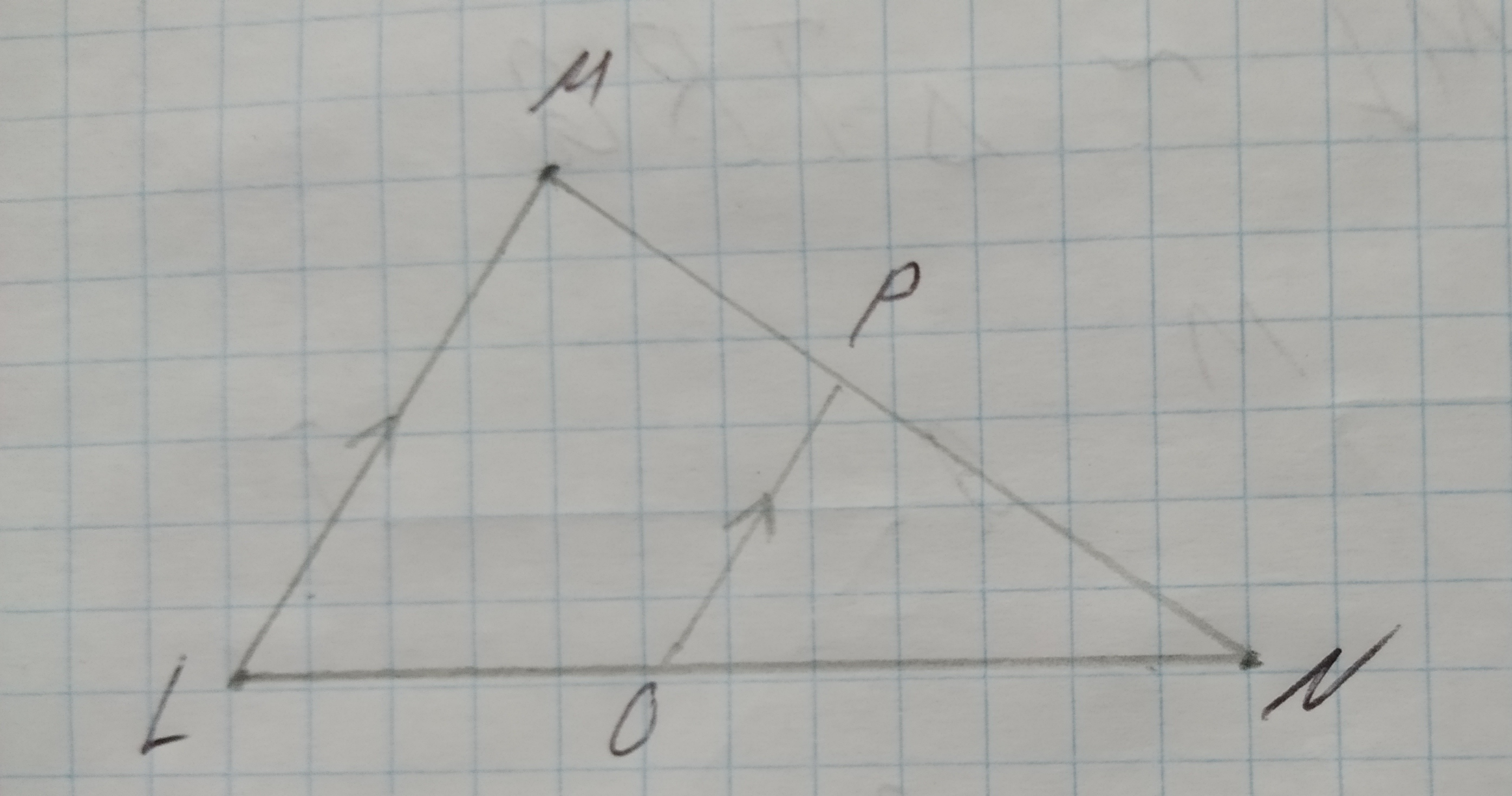Whether the triangle are similar. if so write the similarity statement and if not what would be the sufficient to prove the triangle similar.
Given: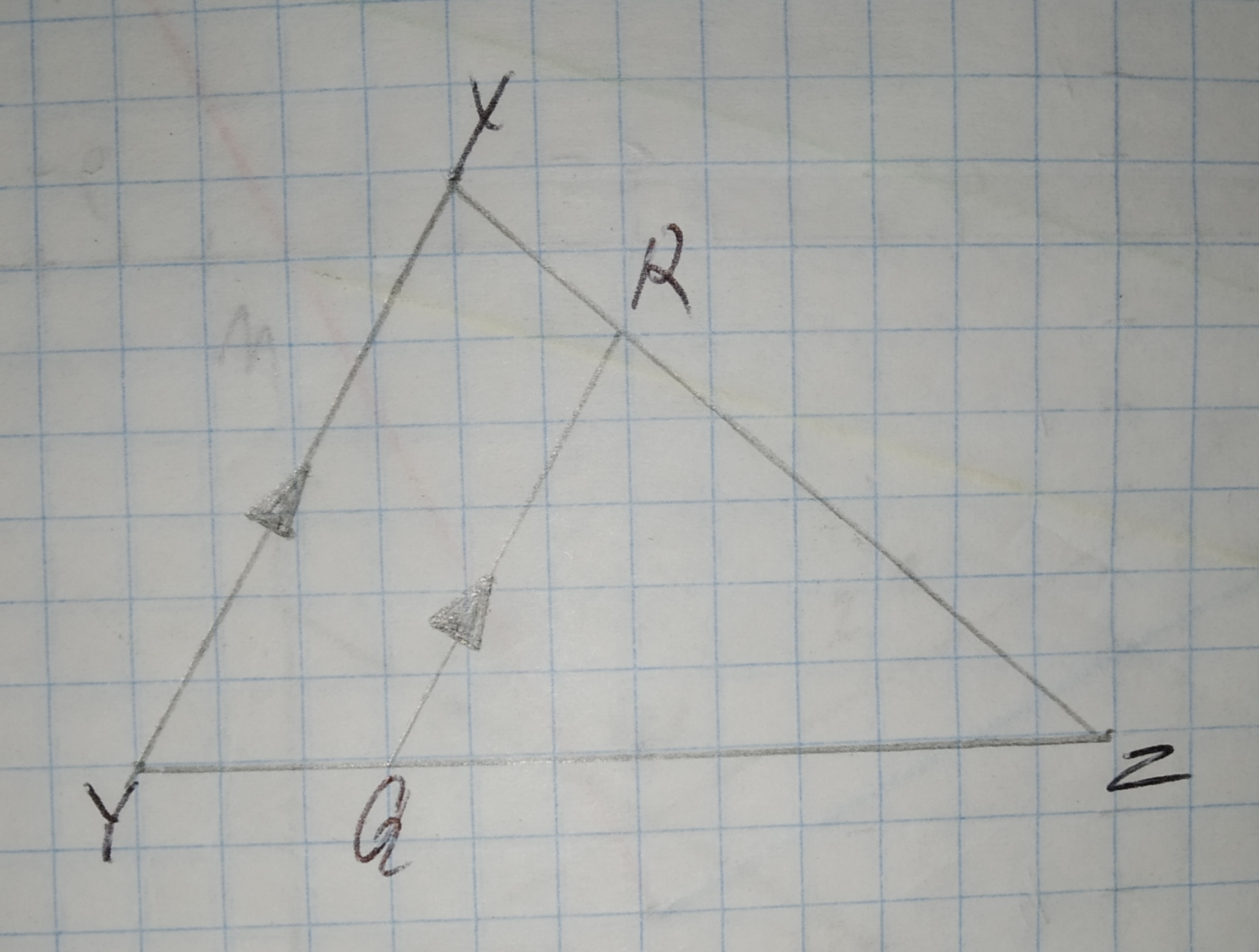Using Similarity Express x in terms of a, b, and c.
Given:
The given pair of triangles is similar.
The given pair of triangle is,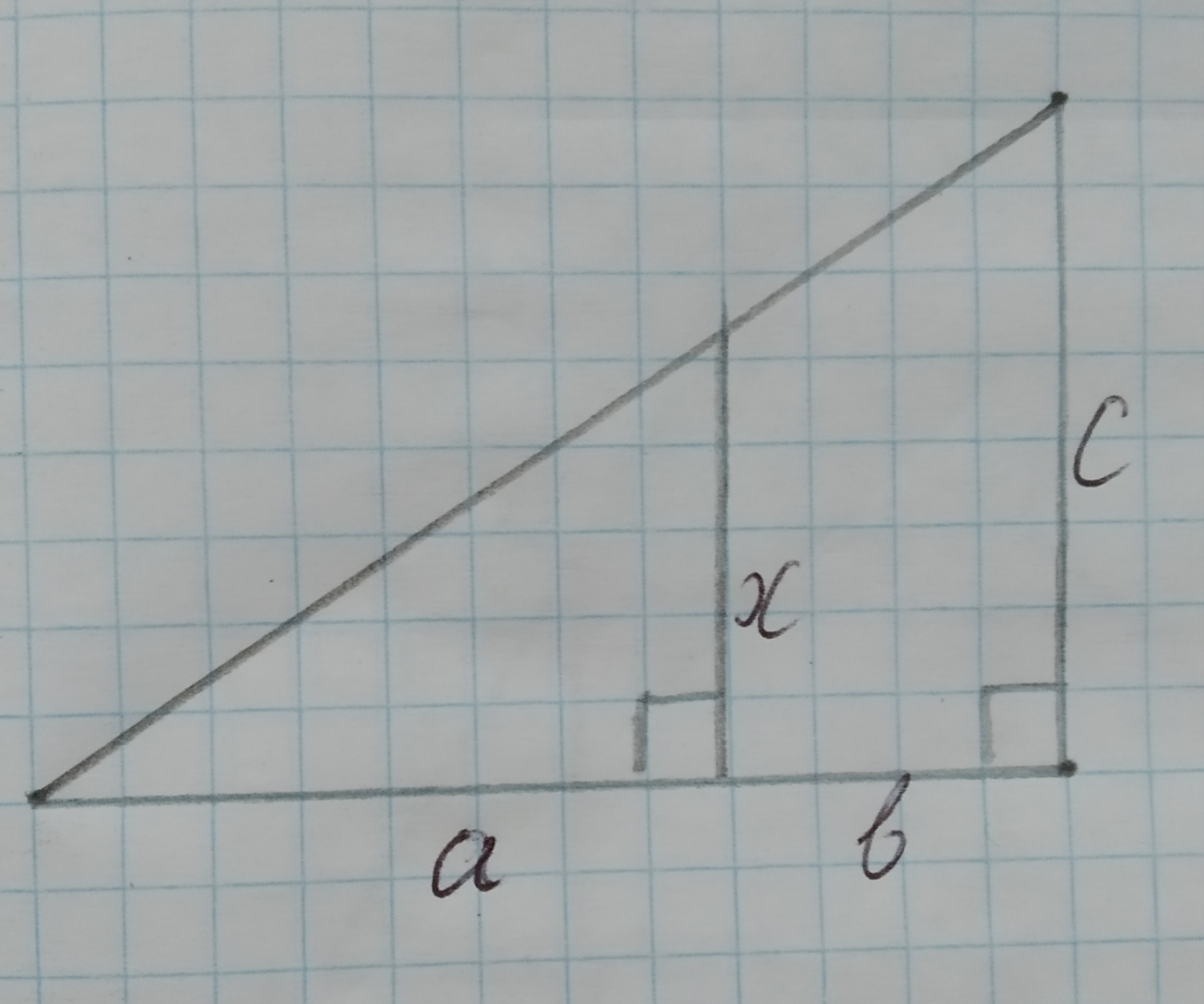To determine:Whether each pair of figures is similar and also write similarity statement and scale factor.
Given information:
Given the figure.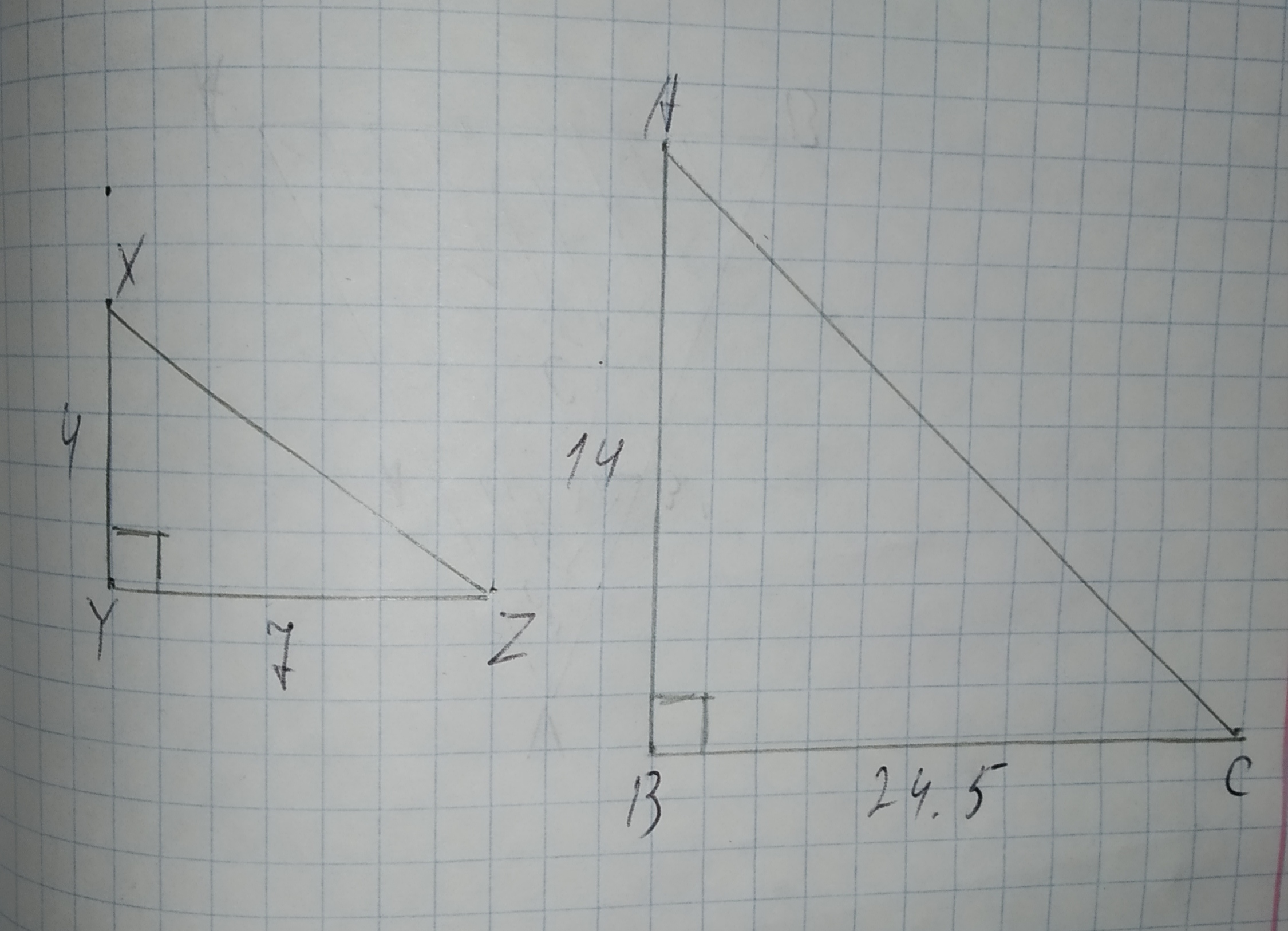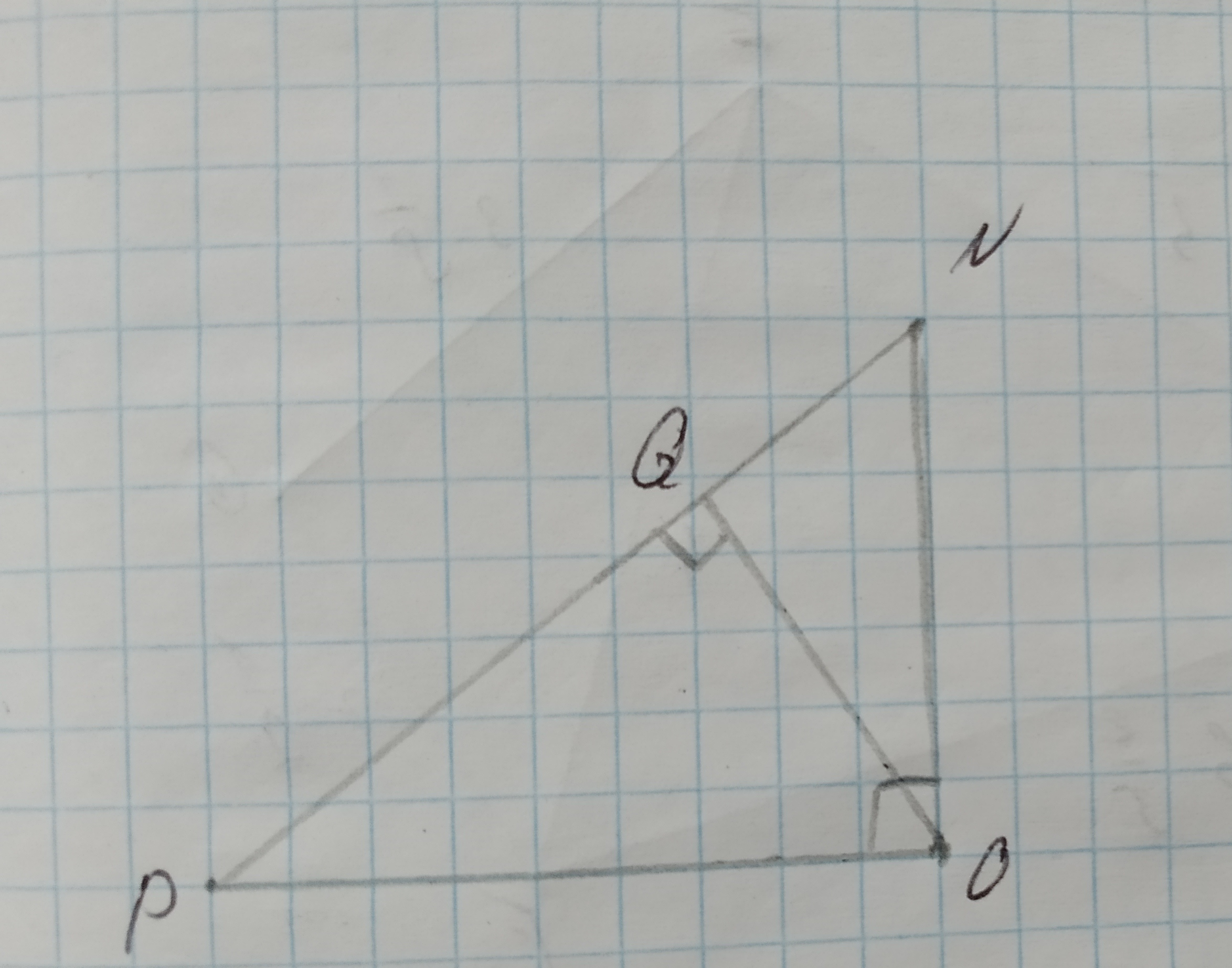To determine:To prove: $$\displaystyle\triangle{R}{S}{T}\sim\triangle{X}{Y}{Z}$$ by the SAS similarity theorem.
$$\displaystyle\angle{R}=\angle{X}$$ (Given)What does similarity imply about the ratios $$\displaystyle\frac{{{B}{C}}}{{{A}{B}}},\frac{{{D}{E}}}{{{A}{D}}},{\quad\text{and}\quad}\frac{{{F}{G}}}{{{A}{F}}}$$?